## A 5.00 mL sample of hydrochloric acid is titrated with 0.1293 M ammonia (a base). If the titration required 28.15 mL of ammonia, determine t

Question

A 5.00 mL sample of hydrochloric acid is titrated with 0.1293 M ammonia (a base). If the titration required 28.15 mL of ammonia, determine the following:
the original concentration of the acid
the original pH of the acid

in progress 0
2 months 2021-08-04T19:20:19+00:00 1 Answers 2 views 0

1. C = 0.73 M.

2. pH = 0.14

Explanation:

The reaction is the following:

HCl + NH₃ ⇄ NH₄⁺Cl⁻

From the titration, we can find the number of moles of HCl that were neutralized by the ammonia.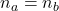Where “a” is for acid and “b” is for base.

The number of moles is: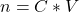Where “C” is for concentration and “V” for volume.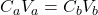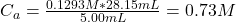Hence the initial concentration of the acid is 0.73 M.

The original pH of the acid is given by: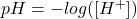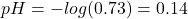Therefore, the original pH of the acid is 0.14.

I hope it helps you!